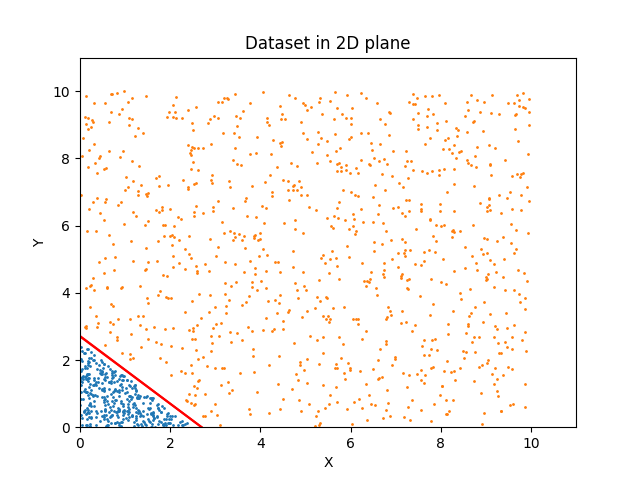# Understanding the learning of sigmoid activations in a neural network

Source: Deep Learning on Medium# Understanding the learning of sigmoid activations in a neural network

In my previous blog, I have explained what the individual neurons in a neural network try to learn. We know that the neurons try to learn classification boundaries by optimizing the weights and biases according to the loss function. Now let’s get to the actual question. What happens after the neurons learn the perfect classification boundary(the case where it exists)? For illustration purpose, let us use a simple example from my previous blog.

In the figure we have the dataset and the neural network that is trying to classify the data. All the learning that the neuron has to do is optimize the weights and biases associated with it to mimic the red classification line.

Now let’s say that we require 25 epochs to learn the exact classification boundary. What if we try to run the model for beyond 25 epochs, let’s say 100 epochs? Is the computation power that we have used for the rest of the 75 epochs worthless? Where did all of this learning go? One might think that once the accuracy reaches 100%(in an ideal scenario), there is nothing to do in the network anymore. Also it is a credible argument because we have reached the global minimum on the loss function curve. But it is not correct. Learning still happens in the network but for a different purpose than optimizing the loss function. To understand this, we must look into some concepts before we jump for explanations.

# What is the neuron in a neural network trying to learn?

As I have explained in my previous blog, each neuron has its own objective of classifying its input based on the feature it is trying to learn. It tries to learn a classification boundary through its weights and biases to assign positive and negative values to points on opposite sides of the line(for detailed explanation I recommend to read by previous blog).